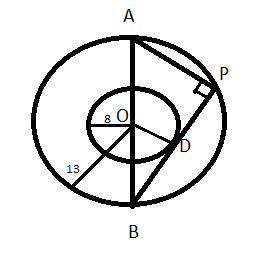QuestionAnswers

# The two concentric circles of radius 13 cm and 8 cm. AB is a diameter of the bigger circle and BD is a tangent to the smaller circle touching it at D and intersecting the larger Circle at P on producing. Find the length of AP.

Hint – In order to solve this problem you need to draw the diagram and analyse it to get the length of AP. Similarity of triangles will play the final role in getting the answer.. Use the property that the diagonal of a circle creates a right angle at its circumference.Above figure is the diagram for this problem. We have joined the common centre O and the point D.
It is given that the two concentric circles of radius 13 cm and 8 cm. AB is a diameter of the bigger circle and BD is a tangent to the smaller circle touching it at D and intersecting the larger Circle at P on producing.
So, we can say from the diagram that AB = 13(2) = 26 (diameter of the larger)
OD = 8 cm (Radius of smaller circle)
OB = 13cm (Radius of larger circle)
We know that the end points of the diameter make a right angle on the circle.
So, we can say that angle APB is 90 degrees.
And we also know that angle ODB is also 90 degrees, since OD is the radius and DB is the tangent.
Therefore on considering the triangles APB and ODB we can say,
$\angle {\text{ABP}} = \angle {\text{OBD}}$(Common angles)
$\angle {\text{APB}} = \angle {\text{ODB}}$ (Right angles)
Therefore, $\Delta {\text{APB}} \sim \Delta {\text{ODB}}$ (By angle-angle similarity criteria)
So, we can say that there respective sides are proportional,
So,
$\dfrac{{{\text{AP}}}}{{{\text{OD}}}}{\text{ = }}\dfrac{{{\text{AB}}}}{{{\text{OB}}}}$
On putting the values we get,
$\dfrac{{{\text{AP}}}}{{\text{8}}}{\text{ = }}\dfrac{{{\text{26}}}}{{{\text{13}}}} \\ {\text{AP = 16cm}} \\$
Hence, the value of AP is 16 cm.

Note – In this problem we have used the concept that if we join the end point of the diameter on the circle then it will construct a right angle triangle right angle on the circle and diameter as hypotenuse. Then we have used the concept that if two triangles are similar then the sides of those triangles are proportional. Proceeding like this will provide you the right answer.